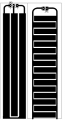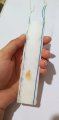# Capacitive soil moisture PCB

#### AlaSH72

Joined Apr 3, 2022
30
hi everyone

I'm about to design a capacitive soil moisture sensor.
I have to make a capacitor on my PCB whose value will change by the change in dielectric, in my case water will effect the dielectric of soil and therefore the value of capacity will change as well.

take a look at this PCB layout which form coplanar capacitycheck this link for a sample of coplanar capacitor PCB as a Capacitive Soil Moisture Sensor

my question is: will really having plates on both sides of PCB give us wider sense of water(moisture)?

here I explain why I think it wont:I made up my own type of capacitor using straight wires held on ruler to keep them well separated in equal distance along.
I used this as my soil moisture capacitor and it did a good job.
then I thought: why not increase the level of water even more? using an other pair of wire on back side?
so I did that too but the result didn't change...
here is a funny thing: both pair wires show equal capacity, but I expect having them both parallel in circuit will give me value 2 times of one pair.
why is that?

#### ApacheKid

Joined Jan 12, 2015
1,094
There is more than capacitance at play here. If the wires are in any way exposed then there's a resistive component, a capacitor with leakage.

How are you measuring the capacitance and accounting for the fact that possibly significant leakage will be present? There are two quantities basically a DC resistance (leakage) and capacitance.

#### AlaSH72

Joined Apr 3, 2022
30
There is more than capacitance at play here. If the wires are in any way exposed then there's a resistive component, a capacitor with leakage.

How are you measuring the capacitance and accounting for the fact that possibly significant leakage will be present? There are two quantities basically a DC resistance (leakage) and capacitance.
what do you mean by "exposed"? wire have thick covers, unless you mean end tips, right?

the way I use to measure capacitance is like so:
I generate a 8MHz square wave using a microcontroller. then I pass this through a Low pass filter consist of a resistor and this made up capacitor.
after that I pass the signal through a peak detector and finally I measure the voltage using a multimeter.
using equation Vc=Vs(1-exp((-62.5*10^-9)/(RC))) where R is known, I figure out C value

#### ApacheKid

Joined Jan 12, 2015
1,094
what do you mean by "exposed"? wire have thick covers, unless you mean end tips, right?

the way I use to measure capacitance is like so:
I generate a 8MHz square wave using a microcontroller. then I pass this through a Low pass filter consist of a resistor and this made up capacitor.
after that I pass the signal through a peak detector and finally I measure the voltage using a multimeter.
using equation Vc=Vs(1-exp((-62.5*10^-9)/(RC))) where R is known, I figure out C value
Yes, I did mean the ends, if there is exposed metal in contact with the earth/water then that resistance must be considered. How does the equation look if we add a leakage resistor across the capacitor plates? (or we can just eliminate the resistance entirely and ensure everything is insulated).

#### AlaSH72

Joined Apr 3, 2022
30
Yes, I did mean the ends, if there is exposed metal in contact with the earth/water then that resistance must be considered. How does the equation look if we add a leakage resistor across the capacitor plates? (or we can just eliminate the resistance entirely and ensure everything is insulated).
I'm not really sure. mentioning this I noticed that my implemented Capacitor's end IS actually exposed to water. but I do not know how leakage resistor takes action in this setup...
nevertheless, any ideas why paralleling both sides capacitors doesn't change anything?

#### ApacheKid

Joined Jan 12, 2015
1,094
I'm not really sure. mentioning this I noticed that my implemented Capacitor's end IS actually exposed to water. but I do not know how leakage resistor takes action in this setup...
nevertheless, any ideas why paralleling both sides capacitors doesn't change anything?
How are the wires paired? did you begin with the blue wire and green wire first (one of the capacitors) and then add the two striped wires to form another capacitor? how are these wired to the circuit?

#### AlaSH72

Joined Apr 3, 2022
30
How are the wires paired? did you begin with the blue wire and green wire first (one of the capacitors) and then add the two striped wires to form another capacitor? how are these wired to the circuit?
as you can see in picture, the first capacitor is made of blue and white/blue wire.
the second capacitor is at back, which is made of green and white/green wire.(you can see that slightly in picture)
so for putting them in parallel in circuit, I connected both green and blue wire together and both white wires together.

#### ApacheKid

Joined Jan 12, 2015
1,094
as you can see in picture, the first capacitor is made of blue and white/blue wire.
the second capacitor is at back, which is made of green and white/green wire.(you can see that slightly in picture)
so for putting them in parallel in circuit, I connected both green and blue wire together and both white wires together.
My first reaction then is that the increase in surface area (two wires as opposed to one) is so small relative to the distance between them, that the impact on the capacitance is minor. What is the measured value? I mean using a capacitance meter?

If we say the wire is 0.5 mm diameter and 15 cm long and the distance is 3 cm we get a capacitance of aprox:

0.0025 * ε

for air ε has value about 9 x 10^-12. giving an approx capacitance (one pair of wires) of 0.0025 pF, very small indeed.

My digital bench multimeter a Siglent SDM3055 can only measure capacitcance down to about 2 nF or 2,000 pF.

This suggest - at first pass - that you'd need to know the resistance very accurately indeed to resolve such a small capacitance value.

I might easily have slipped a decimal place or two so don't take my word for the above, but getting an estimate of what the capacitance should be is a good idea and compare that with what you're calculating.

Last edited: